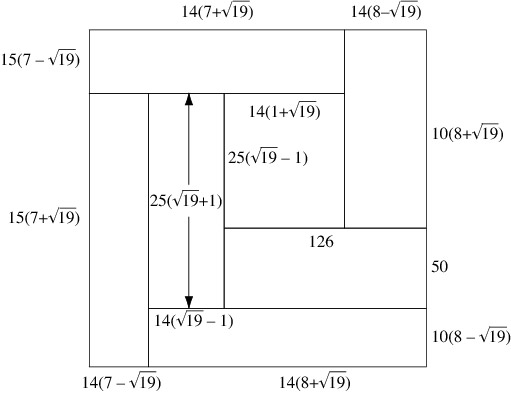A# squareBlanche's dissection.

A square is a quadrilateral with four equal sides that meet at right angles.

To square something is to multiply it by itself. To take the square root is the reverse process. A square number is the square of a whole number. A well known formula for the difference of squares is a2 - b2 = (a + b)(a - b). This formula enables some otherwise difficult calculations to be done easily in the head. For example, 43 × 37 = (40 + 3)(40 - 3) = 402 - 32 = 1600 - 9 = 1591.

Squaring a whole number (integer) gives an integer result, but taking the square root of a whole number often does not. And, as the Greek mathematician Pythagoras and his coworkers discovered, there is not always a rational number expressible as the ratio of two integers) that when squared will equal a particular integer. The square root of 4 is 2 (both integers), but the square root of 2 is somewhere between 1.4142 and 1.4143. The square root of 2 cannot be computed exactly and is called an irrational number.

## Blanche's dissection

Blanche's dissection is the simplest dissection of a square into rectangles of the same areas but different shapes. It is composed of seven pieces; the square is 210 units on a side, and each rectangle has an area of 2102/7 = 6300.